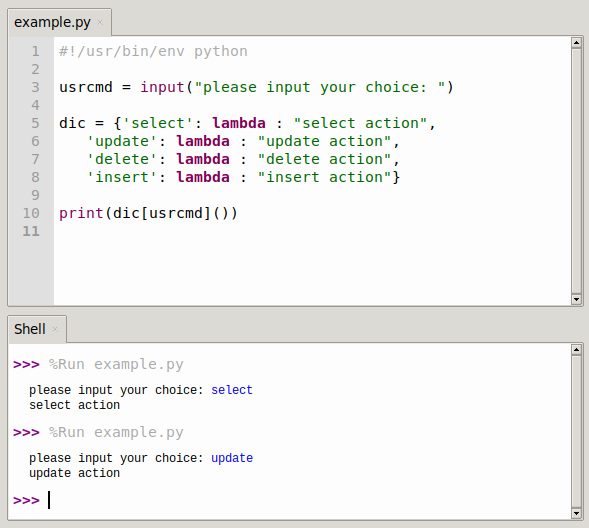# Python switch case

Python does not have the syntax of switch. So the question arose as to how this could be done in python.

In Python the switch-case statement simply doesn’t exist, but that doesn’t mean that’s the end of it.

So how can you use switch in Python, that is known in Java and others?

## Switch case

### Simple if-else

As we know, there are if statements in python, and when learning C, the alternative to if-else is switch, and the two can perfectly replace each other, note that in python else if is simplified to elif, as follows.

``````#!/usr/bin/env python

if usercmd == "select"
ops = "select"
elif usercmd == "update"
ops = "update"
elif usercmd == "delete"
ops = "delete"
elif usercmd == "insert"
ops = "insert"
else
ops = "invalid choice!"
print(ops)``````### Use dictionaries

Here we use the dictionary function: dict.get(key, default=None). key - the value to be looked up in the dictionary, default - returns the default value if the specified key does not exist. As follows.

``````#!/usr/bin/env python

dic = {'select':'select action','update':'update action','delete':'delete action','insert':'insert action'}
defaultitem = 'invalid choice!'
ops = dic.get(usercmd,defaultitem)
print(ops)``````

### Use lambda function combined with dictionary

The general form of lambda is the keyword lambda followed by one or more arguments, followed by a colon, followed by an expression. lambda is an expression and not a statement. It can appear where Python syntax doesn’t allow def to appear, so it won’t be described here much. As follows.

``````#!/usr/bin/env python